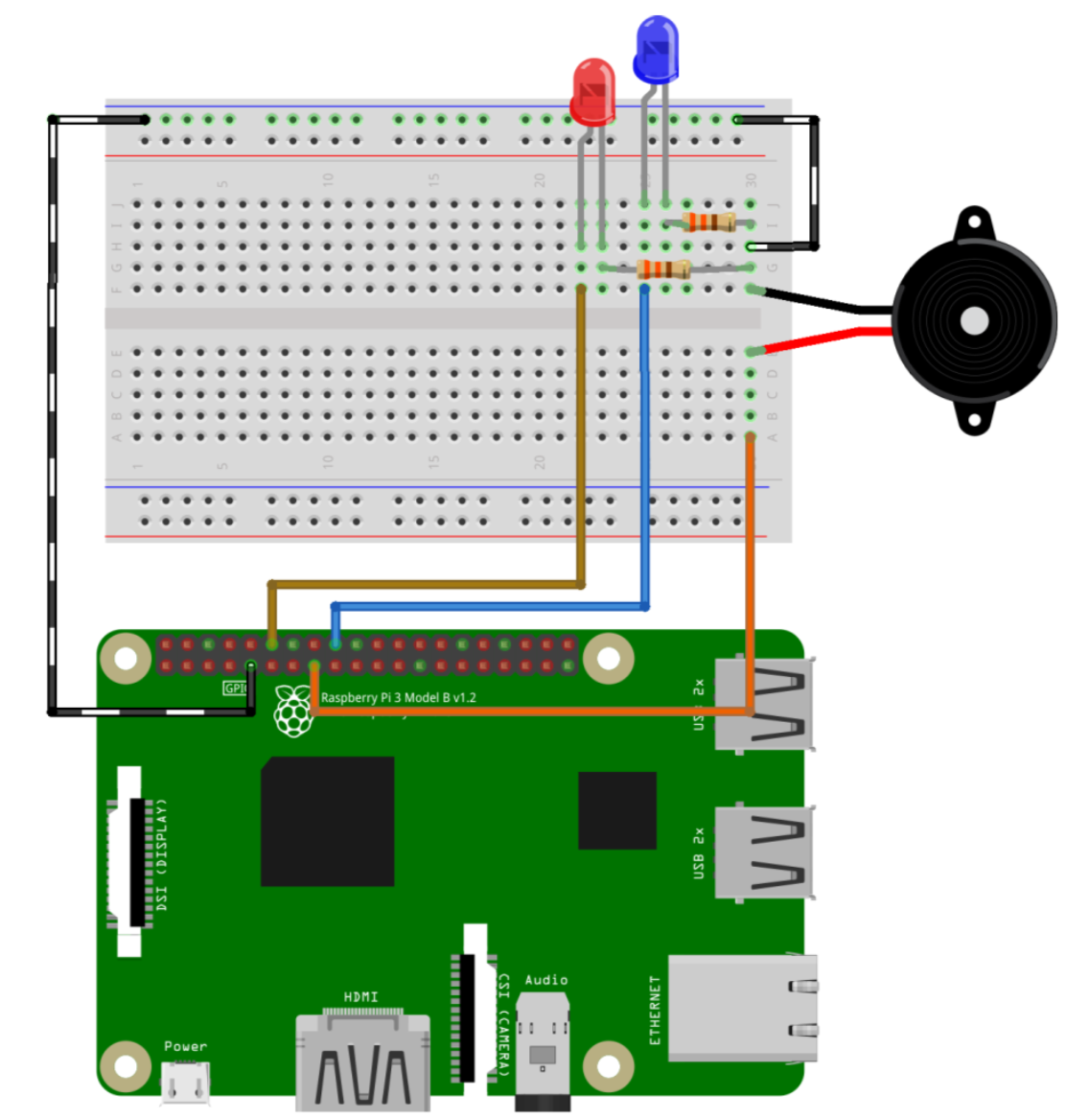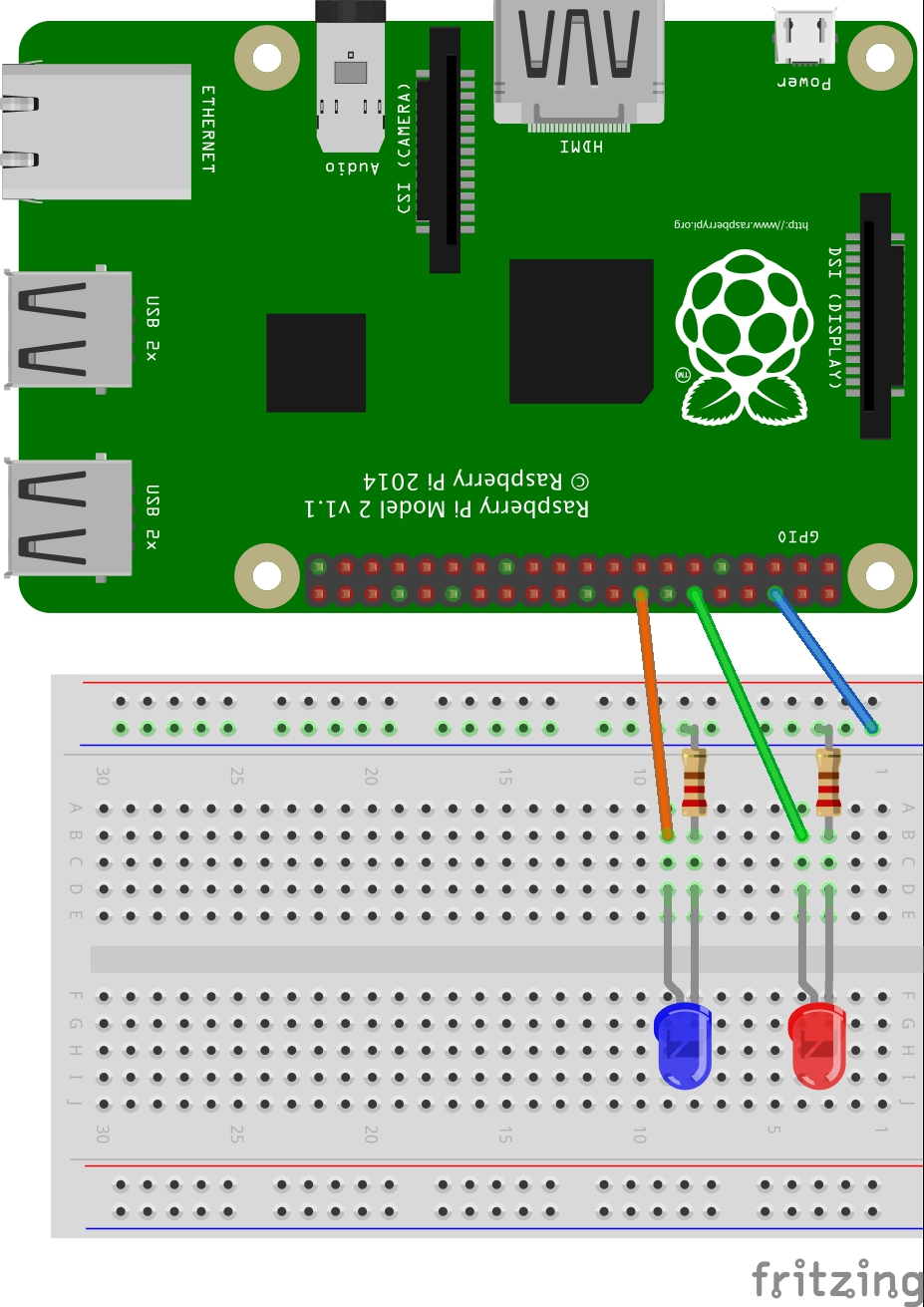NO TIME TO DANCE

RASPBERRY PI

KõlarSTONERIDGE LÜLITI

SONIC PI

Olemasolevate helifailide mängimine

``````3.times do
sample :ambi_sauna
sleep 10
sample :elec_bell
sleep 6
sample :bass_hit_c
sleep 2
end``````

Juhuslikud toonid

``````play rrand(50, 100)

10.times do
play rrand(50, 100)
sleep 0.5
end``````

TÄHEKE

TARK LINN

ROBOTITE SUMO

Sumo 3kg

Edison

ARDUINO

Mootorid

``````//Arduino DC motor controlling

#define motor_pin 3

void setup() { //setup function
// put your setup code here, to run once:
Serial.begin(9600);
Serial.println("Arduino Motor controller");

pinMode(motor_pin, OUTPUT);
}

void loop() { //loop function
// put your main code here, to run repeatedly:
Serial.println("Motor ON");
digitalWrite(motor_pin, HIGH); //turn ON motor

delay(1000); //wait 1second

Serial.println("Motor 0FF");
digitalWrite(motor_pin, LOW); //turn OFF motor

delay(1000); //wait 1second
}``````

ROBOTEX EELVÕISTLUS 04.11.2022

On hea rõõm teatada, et meie meeskond The Beast võitis Lego sumo eelvõistluse kuni 13.aastaste vanusegrupis ja pääseb osalema Robotex International võistlusel.

Suurimad õnnesoovid Karl Kasparile ja Hendrik-Johanile!

SPHERO

Raja läbimine ja topside püüdmine

RASPBERRY PI

LED lambi ühendamine

LED lamp ühendada GND (3.pin) ja GPIO18 (6.pin)
Teine LED lamp ühendada GPIO23 (8.pin)
Lambi lühem jalg läheb takisti pooleKOBRAS viktoriin

https://viktoriinid.ee

PYTHON

Programmeerimine

Lahenda erinevaid ülesandeid kasutades Thonny programmi.

pj4t-modules.eu/et/

## Nutikas Manta ringitund 28.05.18

HOVERBOARD NAGU AUTO

LENDAV SEGWAY

KAHOOTRASPBERRY PI

Esimene tutvus Raspberry PI’gaLIBLIKA ROBOT## TÜG vanemate ringitund 16.05.18

ARDUINO

Servo motorRASPBERRY SENSE HAT

Värviline Digipurkprojects.raspberrypi.org/en/projects/getting-started-with-the-sense-hat

RGB värvid

 255 0 0 Punane 0 255 0 Roheline 0 0 255 Sinine 255 255 0 Kollane 255 0 255 Lilla 0 255 255 Siniroheline

www.w3schools.com/colors/colors_picker.asp?colorhex=ff00ff

RGB kalkulaator

www.w3schools.com/colors/colors_rgb.asp

Tekst

from sense_hat import SenseHat
sense = SenseHat()
sense.show_message(“Hello world”)

RGB värv

from sense_hat import SenseHat
sense = SenseHat()
r = 255
g = 255
b = 255
sense.clear((r, g, b))

Liikuv tekst

from sense_hat import SenseHat
sense = SenseHat()
blue = (0, 0, 255)
yellow = (255, 255, 0)
sense.show_message(“Astro Pi is awesome!”, text_colour=yellow, back_colour=blue, scroll_speed=0.05)

Erinevat värvi tähed

from sense_hat import SenseHat
from time import sleep
sense = SenseHat()
red = (255, 0, 0)
blue = (0, 0, 255)
green = (0, 255, 0)
white = (255, 255, 255)
yellow = (255, 255, 0)
sense.show_letter(“L”, red)
sleep(1)
sense.show_letter(“a”, blue)
sleep(1)
sense.show_letter(“u”, green)
sleep(1)
sense.show_letter(“r”, white)
sleep(1)
sense.show_letter(“a”, yellow)

Suvalised värvid

from sense_hat import SenseHat
from time import sleep
from random import randint
sense = SenseHat()
red = (255, 0, 0)
blue = (0, 0, 255)
green = (0, 255, 0)
white = (255, 255, 255)
yellow = (255, 255, 0)
# Generate a random colour
def pick_random_colour():
random_red = randint(0, 255)
random_green = randint(0, 255)
random_blue = randint(0, 255)
return (random_red, random_green, random_blue)
sense.show_letter(“L”, pick_random_colour())
sleep(1)
sense.show_letter(“a”, pick_random_colour())
sleep(1)
sense.show_letter(“u”, pick_random_colour())
sleep(1)
sense.show_letter(“r”, pick_random_colour())
sleep(1)
sense.show_letter(“a”, pick_random_colour())
sleep(1)
sense.clear()

Pikslid

from sense_hat import SenseHat
sense = SenseHat()
blue = (0, 0, 255)
red = (255, 0, 0)
sense.set_pixel(0, 2, blue)
sense.set_pixel(7, 4, red)

from sense_hat import SenseHat
sense = SenseHat()
sense.set_pixel(2, 2, (0, 0, 255))
sense.set_pixel(4, 2, (0, 0, 255))
sense.set_pixel(3, 4, (100, 0, 0))
sense.set_pixel(1, 5, (255, 0, 0))
sense.set_pixel(2, 6, (255, 0, 0))
sense.set_pixel(3, 6, (255, 0, 0))
sense.set_pixel(4, 6, (255, 0, 0))
sense.set_pixel(5, 5, (255, 0, 0))

from sense_hat import SenseHat
sense = SenseHat()
# Define some colours
g = (0, 255, 0) # Green
b = (0, 0, 0) # Black
# Set up where each colour will display
creeper_pixels = [
g, g, g, g, g, g, g, g,
g, g, g, g, g, g, g, g,
g, b, b, g, g, b, b, g,
g, b, b, g, g, b, b, g,
g, g, g, b, b, g, g, g,
g, g, b, b, b, b, g, g,
g, g, b, b, b, b, g, g,
g, g, b, g, g, b, g, g
]
# Display these colours on the LED matrix
sense.set_pixels(creeper_pixels)

Õhurõhk

from sense_hat import SenseHat
sense = SenseHat()
sense.clear()
pressure = sense.get_pressure()
print(pressure)

Temperatuur

from sense_hat import SenseHat
sense = SenseHat()
sense.clear()
temp = sense.get_temperature()
print(temp)

Õhuniiskus

from sense_hat import SenseHat
sense = SenseHat()
sense.clear()
humidity = sense.get_humidity()
print(humidity)

Õhurõhk, temperatuur ja õhuniiskus

from sense_hat import SenseHat
sense = SenseHat()
while True:
# Take readings from all three sensors
t = sense.get_temperature()
p = sense.get_pressure()
h = sense.get_humidity()
# Round the values to one decimal place
t = round(t, 1)
p = round(p, 1)
h = round(h, 1)
# Create the message
# str() converts the value to a string so it can be concatenated
message = “Temperature: ” + str(t) + ” Pressure: ” + str(p) + ” Humidity: ” + str(h)
# Display the scrolling message
sense.show_message(message, scroll_speed=0.05)

from sense_hat import SenseHat
sense = SenseHat()
# Define the colours red and green
red = (255, 0, 0)
green = (0, 255, 0)
while True:
# Take readings from all three sensors
t = sense.get_temperature()
p = sense.get_pressure()
h = sense.get_humidity()
# Round the values to one decimal place
t = round(t, 1)
p = round(p, 1)
h = round(h, 1)
# Create the message
# str() converts the value to a string so it can be concatenated
message = “Temperature: ” + str(t) + ” Pressure: ” + str(p) + ” Humidity: ” + str(h)
if t > 18.3 and t < 26.7:
bg = green
else:
bg = red
# Display the scrolling message
sense.show_message(message, scroll_speed=0.05, back_colour=bg)

Liikuminefrom sense_hat import SenseHat
sense = SenseHat()
sense.clear()
o = sense.get_orientation()
pitch = o[“pitch”]
roll = o[“roll”]
yaw = o[“yaw”]
print(“pitch {0} roll {1} yaw {2}”.format(pitch, roll, yaw))

from sense_hat import SenseHat
sense = SenseHat()
while True:
acceleration = sense.get_accelerometer_raw()
x = acceleration[‘x’]
y = acceleration[‘y’]
z = acceleration[‘z’]
x=round(x, 0)
y=round(y, 0)
z=round(z, 0)
print(“x={0}, y={1}, z={2}”.format(x, y, z))

from sense_hat import SenseHat
sense = SenseHat()
# Display the letter J
sense.show_letter(“J”)
while True:
acceleration = sense.get_accelerometer_raw()
x = acceleration[‘x’]
y = acceleration[‘y’]
z = acceleration[‘z’]
x=round(x, 0)
y=round(y, 0)
z=round(z, 0)
print(“x={0}, y={1}, z={2}”.format(x, y, z))
# Update the rotation of the display depending on which way up the Sense HAT is
if x == -1:
sense.set_rotation(180)
elif y == 1:
sense.set_rotation(90)
elif y == -1:
sense.set_rotation(270)
else:
sense.set_rotation(0)

Joystick – klaviatuuri noolte kasutamine

from sense_hat import SenseHat
sense = SenseHat()
while True:
for event in sense.stick.get_events():
print(event.direction, event.action)

from sense_hat import SenseHat
from time import sleep
sense = SenseHat()
e = (0, 0, 0)
w = (255, 255, 255)
sense.clear()
while True:
for event in sense.stick.get_events():
# Check if the joystick was pressed
if event.action == “pressed”:
# Check which direction
if event.direction == “up”:
sense.show_letter(“U”) # Up arrow
elif event.direction == “down”:
sense.show_letter(“D”) # Down arrow
elif event.direction == “left”:
sense.show_letter(“L”) # Left arrow
elif event.direction == “right”:
sense.show_letter(“R”) # Right arrow
elif event.direction == “middle”:
sense.show_letter(“M”) # Enter key
# Wait a while and then clear the screen
sleep(0.5)
sense.clear()

from sense_hat import SenseHat
sense = SenseHat()
# Define the functions
def red():
sense.clear(255, 0, 0)
def blue():
sense.clear(0, 0, 255)
def green():
sense.clear(0, 255, 0)
def yellow():
sense.clear(255, 255, 0)
# Tell the program which function to associate with which direction
sense.stick.direction_up = red
sense.stick.direction_down = blue
sense.stick.direction_left = green
sense.stick.direction_right = yellow
sense.stick.direction_middle = sense.clear # Press the enter key
while True:
pass # This keeps the program running to receive joystick events

Projekt – reaktsiooni kiiruse mäng

# IMPORT the required libraries (sense_hat, time, random)
from sense_hat import SenseHat
from time import sleep
from random import choice
# CREATE a sense object
sense = SenseHat()
# Set up the colours (white, green, red, empty)
w = (150, 150, 150)
g = (0, 255, 0)
r = (255, 0, 0)
e = (0, 0, 0)
# Create images for three different coloured arrows
arrow = [
e,e,e,w,w,e,e,e,
e,e,w,w,w,w,e,e,
e,w,e,w,w,e,w,e,
w,e,e,w,w,e,e,w,
e,e,e,w,w,e,e,e,
e,e,e,w,w,e,e,e,
e,e,e,w,w,e,e,e,
e,e,e,w,w,e,e,e
]
arrow_red = [
e,e,e,r,r,e,e,e,
e,e,r,r,r,r,e,e,
e,r,e,r,r,e,r,e,
r,e,e,r,r,e,e,r,
e,e,e,r,r,e,e,e,
e,e,e,r,r,e,e,e,
e,e,e,r,r,e,e,e,
e,e,e,r,r,e,e,e
]
arrow_green = [
e,e,e,g,g,e,e,e,
e,e,g,g,g,g,e,e,
e,g,e,g,g,e,g,e,
g,e,e,g,g,e,e,g,
e,e,e,g,g,e,e,e,
e,e,e,g,g,e,e,e,
e,e,e,g,g,e,e,e,
e,e,e,g,g,e,e,e
]
# Set a variable pause to 3 (the initial time between turns)
# Set variables score and angle to 0
# Create a variable called play set to True (this will be used to stop the game later)
pause = 3
score = 0
angle = 0
play = True
sense.show_message(“Keep the arrow pointing up”, scroll_speed=0.05, text_colour=[100,100,100])
# WHILE play == True
while play:
# CHOOSE a new random angle
last_angle = angle
while angle == last_angle:
angle = choice([0, 90, 180, 270])
sense.set_rotation(angle)
# DISPLAY the white arrow
sense.set_pixels(arrow)
# SLEEP for current pause length
sleep(pause)
acceleration = sense.get_accelerometer_raw()
x = acceleration[‘x’]
y = acceleration[‘y’]
z = acceleration[‘z’]
x = round(x, 0)
y = round(y, 0)
print(angle)
print(x)
print(y)
# IF orientation matches the arrow…
if x == -1 and angle == 180:
# ADD a point and turn the arrow green
sense.set_pixels(arrow_green)
score += 1
elif x == 1 and angle == 0:
sense.set_pixels(arrow_green)
score += 1
elif y == -1 and angle == 90:
sense.set_pixels(arrow_green)
score += 1
elif y == 1 and angle == 270:
sense.set_pixels(arrow_green)
score += 1
else:
# SET play to `False` and DISPLAY the red arrow
sense.set_pixels(arrow_red)
play = False
# Shorten the pause duration slightly
pause = pause * 0.95
# Pause before the next arrow
sleep(0.5)
# When loop is exited, display a message with the score
msg = “Your score was %s” % score
sense.show_message(msg, scroll_speed=0.05, text_colour=[100, 100, 100])

Veel ideid

• Tell a joke on the LED screen.
• If your Sense HAT is connected to the internet, you could use a Twitter API library to make it display incoming tweets.
• Create your own images to display on the LED matrix.
• Can you alternate between images to create an animation? Check out this Geek Gurl Diaries video for some inspiration.
• Create an electronic dielike this one. Shaking the Pi triggers the roll of the die.
• Create a simple graphical thermometer which outputs different colours or patterns depending on the temperature.
• Write a program that displays an arrow (or other symbol) on screen; this symbol could be used to point to which way is down. This way, astronauts in low gravity always know where the Earth is.
• Use the accelerometer to sense small movements — this could form part of a game, alarm system, or even an earthquake sensor.
• Make use of the humidity sensor to detect breath and display a colour depending on the humidity.

## TÜG nooremate ringitund 08.05.18

Interaktiivne bowling

Transformer robot

SCRATCH

Speaker

Näide, kuidas kasutada spraidi kõlarit ja sellega muusikat teha._Melody =

10-.125/9.5-.125/10-.125/9.5-.125/10-.125/7-.125/9-.125/8-.125/6-.375/1-.125/3-.125/6-.125/7-.375/3-.125/5.5-.125/7-.125/8-.375/3-.125/10-.125/9.5-.125/10-.125/9.5-.125/10-.125/7-.125/9-.125/8-.125/6-.375/1-.125/3-.125/6-.125/7-.375/3-.125/8-.125/7-.125/6-.375/7-.125/8-.125/9-.125/10-.375/5-.125/11-.125/10-.125/9-.375/4-.125/10-.125/9-.125/8-.375/3-.125/9-.125/8-.125/7-.375/3-.125/10-.125/9.5-.125/10-.125/9.5.125/10-.125/7-.125/9-.125/8-.125/6-.375/1-.125/3-.125/6-.125/7-.375/3-.125/8-.125/7-.125/6-.5/

Sound

Kõigepealt tuleb teha valmis spraidid erinevate kujunditega. Igal spraidil on erinev arv ruute, mis siis hiljem vahelduma hakkavad.Spraid kood.CUBROIDwww.cubroid.com

codingblocks.cubroid.com

Materjalid

Cubroid raamat

Cubroid juhendRASPBERRY HAT

Minecrafti moodi